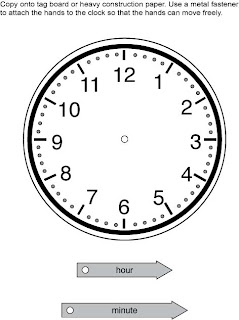## Monday, June 4, 2012

### Time for Analog Clocks

In Excel Math, students learn to tell time using analog and digital clocks.
A clock with a minute and an hour hand is called analog. An analog clock has hands to point out the time:

Here's a cool analog chalkboard watch our friend Mike brought back from a recent trip to England. He can even customize the face with his own piece of chalk:

digital clock has only digits to the indicate the time (no hands):

Bring an analog clock with movable hands to your class, or make one using this analog clock from Excel Math First Grade Manipulative M14. Cut out the hands and attach them to the clock face with a paper fastener:Analog Clock from Excel Math First Grade Teacher Edition M14Click here to download the PDF file.
Show the students your clock. Explain that the shorter (usually fatter) hand indicates the hour and the longer hand indicates the number of minutes after the hour. If the minute hand is pointing straight up, the time is on the hour with zero minutes.

Display 3:00 on your clock. Show it to the students and ask them what time it is. Point out the face and the hands of the clock. Explain that as the minute hand moves around the clock face, the hour hand will gradually move to the next hour mark. Show how this happens by moving the hands around the clock to show 4:00.

Explain that the hour hand points directly at a numeral only when it is exactly on that hour. The rest of the time the hour hand will be pointing in between two numerals.

Point out the minute marks on your clock. Have a student count the marks. Ask the class if there is a pattern in the number of minute marks between each of the hour marks. (There are five minute marks between every two hour marks.)

Set your clock to 4:25. Show the students the clock face and ask them what time it is. Ask them how they might calculate the minutes after the hour. (They can count each minute mark or count by five.)

Repeat this process several times where the minute hand points to a multiple of five.

Next, set your clock to 4:27. Ask the students what time it is.  Ask them how they might calculate the minutes after the hour. (The can count each minute mark or count by five until they get close to the minute hand and then they can count each minute mark.) Repeat this process several times where the minute hand points to a number that is not a multiple of five.

Print the following Excel Math worksheet for your students. Let them complete numbers 1-6 on their own. Offer help as needed:Excel Math Third Grade Student Lesson Worksheet #18 Click here to download the PDF file

Read more about analog clocks on our March 29 blog post. Visit the Excel Math website for more lesson ideas.